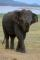# Percent

From 99 children who participated in the ski course were 23 excellent skiers, 13 good and 20 average and the rest were beginners.

Calculate this data in percentages.

Result

p1 =  23.2 %
p2 =  13.1 %
p3 =  20.2 %
p4 =  43.4 %

#### Solution:Leave us a comment of example and its solution (i.e. if it is still somewhat unclear...):Be the first to comment!## Next similar examples:

1. The percentages in practiceIf every tenth apple on the tree is rotten it can be expressed by percentages: 10% of the apples on the tree is rotten. Tell percent using the following information: a. in June rained 6 days b, increase worker pay 500 euros to 50 euros c, grabbed 21 fro
2. Percents - easyHow many percent is 432 out of 434?
3. Base, percents, valueBase is 344084 which is 100 %. How many percent is 384177?
4. Conference148 is the total number of employees. The conference was attended by 22 employees. How much is it in percent?
5. GlovesI have a box with two hundred pieces of gloves in total, split into ten parcels of twenty pieces, and I sell three parcels. What percent of the total amount I sold?
6. Apples 2James has 13 apples. He has 30 percent more apples than Sam. How many apples has Sam?
7. TVsProduction of television sets increased from 3,500 units to 4,200 units. Calculate the percentage of production increase.
8. Seeds 2How many seeds germinated from 1000 pcs, when 23% no emergence?
9. ClassIn a class are 32 pupils. Of these are 8 boys. What percentage of girls are in the class?
10. PercentsHow many percents is 900 greater than the number 750?
11. New refrigeratorNew refrigerator sells for 1024 USD, Monday will be 25% discount. How much USD will save, and what will be the price?
12. IronIron ore contains 57% iron. How much ore is needed to produce 20 tons of iron?
13. NumberWhat number is 20 % smaller than the number 198?
14. Highway repairThe highway repair was planned for 15 days. However, it was reduced by 30%. How many days did the repair of the highway last?
15. 100 %How much is 100%, if 17 % is 1169?
16. ClassIn 7.C clss are 10 girls and 20 boys. Yesterday was missing 20% of girls and 50% boys. What percentage of students missing?
17. Sales offGoods is worth € 70 and the price of goods fell two weeks in a row by 10%. How many % decreased overall?